## Simple Truss

In the simple truss below, which members have a zero force?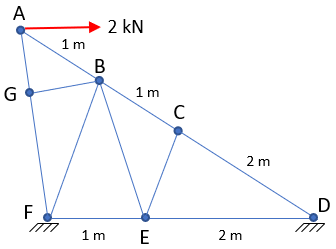Hint
In a truss system, zero force members exist if members are aligned in the below set up as long as there are no direct external loads.Member KB is a zero force member because the forces in members KC and KA are equal and opposite.
Hint 2
To start out simple, let's first analyze Point C and Point G.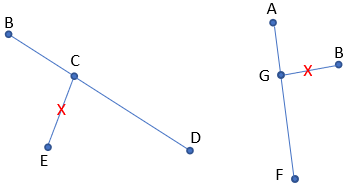Members CE and GB are zero force.
In a truss system, zero force members exist if members are aligned in the below set up as long as there are no direct external loads.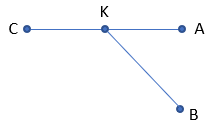Member KB is a zero force member because the forces in members KC and KA are equal and opposite. Member KB can be any arbitrary angle and still be zero force.

To start out simple, let's first analyze Point C and Point G.Members CE and GB are zero force. Next, let's look at Point E.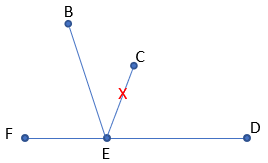Because we previously determined member CE is zero force, member EB must also be a zero force. Finally let's analyze Point B.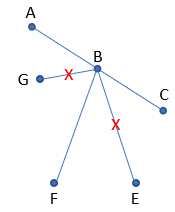Because we previously determined members GB and EB are zero force, member BF must also be a zero force. Thus, members CE, GB, EB, and BF are zero force members.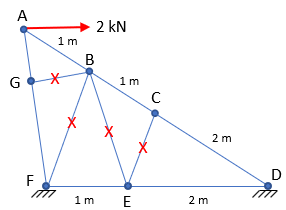Members CE, GB, EB, and BF are zero force members.